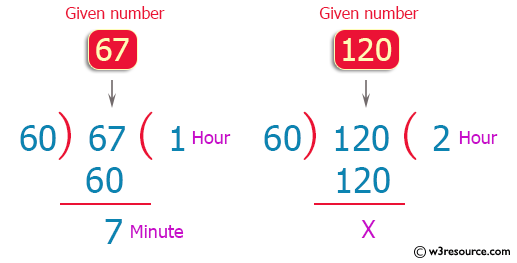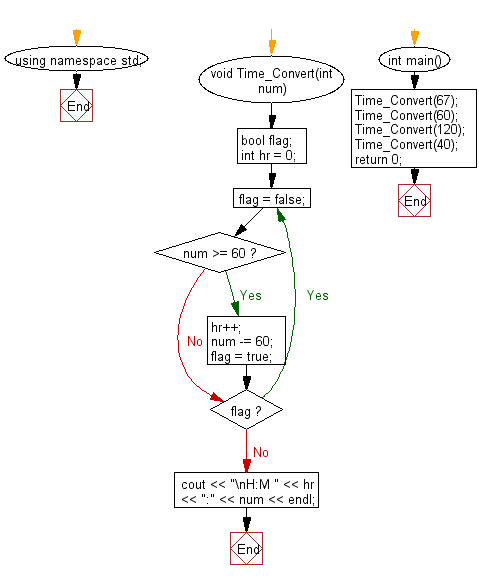﻿ C++ Exercises: Convert a given number into hours and minutes - w3resource

# C++ Exercises: Convert a given number into hours and minutes

## C++ Basic: Exercise-83 with Solution

Write a C++ program to convert a given number into hours and minutes. Separate the number of hours and minutes with a colon.

For example if a given number is 67 the output should be 1:7

Pictorial Presentation:Sample Solution:

C++ Code :

``````#include <iostream>
#include <string>
using namespace std;
void Time_Convert(int num) {
bool flag;
int hr = 0;
do
{
flag = false;
if (num >= 60)
{
hr++;
num -= 60;
flag = true;
}
} while (flag);
cout << "\nH:M " << hr << ":" << num << endl;
}

int main() {
Time_Convert(67);
Time_Convert(60);
Time_Convert(120);
Time_Convert(40);
return 0;
}
``````

Sample Output:

```H:M 1:7

H:M 1:0
```

Flowchart:C++ Code Editor:

What is the difficulty level of this exercise?

﻿

## C++ Programming: Tips of the Day

Why is there no std::stou?

The most pat answer would be that the C library has no corresponding "strtou", and the C++11 string functions are all just thinly veiled wrappers around the C library functions: The std::sto* functions mirror strto*, and the std::to_string functions use sprintf.

Ref: https://bit.ly/3wtz2qA

We are closing our Disqus commenting system for some maintenanace issues. You may write to us at reach[at]yahoo[dot]com or visit us at Facebook